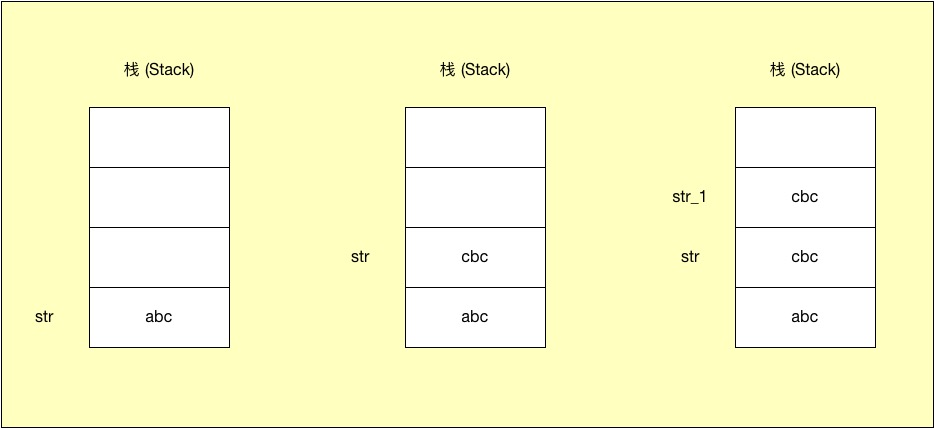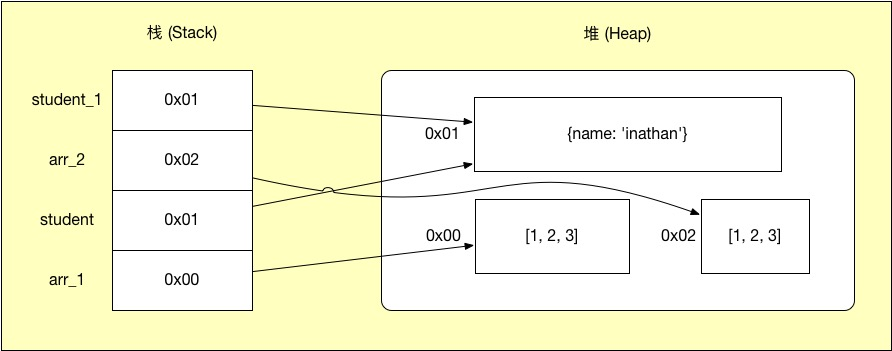# '浅拷贝'、'深拷贝'

July 22, 2019 访问: 2,793 次

## 堆栈

### 基本类型

``````let str = 'abc'
str = 'd'
console.log(str)
// abc
// 通过字符串索引来更改字符串并不会像我们期望的那样改变其原始值
str = 'cbc'
console.log(str)
// cbc
// 只有重新赋值才会改变变量str的值，但是依然无法改变'abc'的原始值
str_1 = str
// 将变量str的值传递给变量str_1````````````let a = 1
let b = 1
console.log(a === b)
// true``````

### 引用类型``````let arr_1 = [1, 2, 3]
let student = {name: 'inathan'}
let arr_2 = [1, 2, 3]
let student_1 = student``````

``````console.log(arr_1 == arr_2)
// false``````

``````arr_1 = 4
console.log(arr_1)
// [4, 2, 3]``````

``````student_1.name = 'wang'
console.log(student.name)
// wang
// 两个变量都指向同一地址，改变其中任何一个变量的值，都会改变另一个变量``````

## 浅拷贝

``````function copy (src) {
// 这里简单写一个只针对对象的浅拷贝，其他类型抛出异常
if (typeof src !== 'object') {
throw new Error('类型错误：没有对象！')
}
// 判断传入参数是数组还是object
let result = src.constructor.name === 'Array' ? [] : {}
// 遍历对象
for (let key in src) {
// 使用src.hasOwnProperty()方法过滤掉src对象中继承在原型链上的属性
if (src.hasOwnProperty(key)) {
result[key] = src[key] // 赋值
}
}
return result
}

let obj_1 = {
'name' : 'wang',
'age' : 29,
'arr' : [1, 2, [3, 4]],
}

let obj_2 = copy(obj_1)

obj_2.name = 'inathan'
obj_2.arr = 5

console.log(obj_1)
//{
//    'name' : 'wang',
//    'age' : 29,
//    'arr' : [5, 2, [3, 4]],
//}

console.log(obj_2)
//{
//    'name' : 'inathan',
//    'age' : 29,
//    'arr' : [5, 2, [3, 4]],
//}``````

es6中的 `...`扩展运算符也是浅拷贝

## 深拷贝

### for...in

``````function deepCopy (src) {
// 这里简单写一个只针对对象的浅拷贝，其他类型抛出异常
if (typeof src !== 'object') {
throw new Error('类型错误：没有对象！')
}
// 判断传入参数是数组还是object
let result = src.constructor.name === 'Array' ? [] : {}
// 遍历对象
for (let key in src) {
// 使用src.hasOwnProperty()方法过滤掉src对象中继承在原型链上的属性
if (src.hasOwnProperty(key)) {
// 如果src子元素是对象，执行递归操作
if (src[key] && typeof src[key] === 'object') {
result[key] = deepCopy(src[key])
} else {
// 不是对象，直接赋值
result[key] = src[key]
}
}
}
return result
}

let obj_1 = {
'name' : 'wang',
'age' : 29,
'arr' : [1, 2, [3, 4]],
}

let obj_2 = deepCopy(obj_1)

obj_2.name = 'inathan'
obj_2.arr = 5
obj_2.arr = 6

console.log(obj_1)
//{
//    'name' : 'wang',
//    'age' : 29,
//    'arr' : [1, 2, [3, 4]],
//}

console.log(obj_2)
//{
//    'name' : 'inathan',
//    'age' : 29,
//    'arr' : [5, 2, [6, 4]],
//}``````

### 序列化反序列化法：

``````function deepCopy (src) {
return JSON.parse(JSON.stringify(src))
}``````

``````function foo () {
this.name = 'wang'
}
foo.prototype.age = 20
let o = new foo()
o_1 = JSON.parse(JSON.stringify(o))
console.log(o_1.age)
// undefined``````

### Reflect对象

``````function deepCopy (src) {
if (typeof src !== 'object') {
throw new Error('类型错误：没有对象！')
}

// 判断传入参数是数组还是object,进行浅拷贝
let result = src.constructor.name === 'Array' ? [...src] : {...src}

// 遍历浅拷贝后key组成的数组
Reflect.ownKeys(result).forEach(key => {
// 判断浅拷贝后的result子元素是否为object类型，如果是，需要再次拷贝，不是无需处理
if (typeof result[key] === 'object') {
result[key] = deepCopy(result[key]) // 子元素是对象，递归操作
}
})

return result
}``````